Classifying FractionsLook at each fraction below. How are these fractions similar? How are they different?The fractions above are similar since each one has a denominator of 4. Look at the circles below to see how these fractions are different.

1/4 (One-fourth)      4/4 (Four-fourths)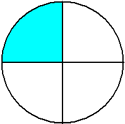7/4 (Seven-fourths)The fraction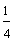is called a proper fraction. The fractions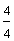andare improper fractions.

Definition: A proper fraction is a fraction in which the numerator is less than the denominator.

Definition: An improper fraction is a fraction in which the numerator is greater than or equal to the denominator.

In example 1, we will identify each fraction as proper or improper. We will also write each fraction using words.

 Example 1 Fraction Type Wordsimproper three-halves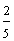proper two-fifthsimproper three-thirdsproper five-sixths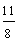improper eleven-eighths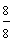improper eight-eighths

What do the fractions in example 2 have in common?

Example 22/2 (Two-halves)3/3 (Three-thirds)4/4 (Four-fourths)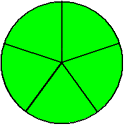5/5 (Five-fifths)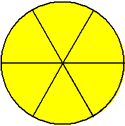6/6 (Six-sixths)

In example 2, each fraction has a numerator that is equal to its denominator. Each of these fractions is an improper fraction, equal to one whole (1). An improper fraction can also be greater than one whole, as shown in example 3.

Example 37/4 (Seven Fourths)

In the improper fraction seven-fourths, the numerator (7) is greater than the denominator (4). We can write this improper fraction as a mixed number.

Definition: mixed number consists of a whole-number part and a fractional part.

In examples 4 through 6, we will write each improper fraction as a mixed number.

Example 4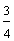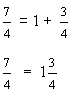In example 4, seven-fourths is an improper fraction. It is really the sum of four-fourths and three-fourths. Seven-fourths is written as the mixed number one and three-fourths, where one is the whole-number part, and three-fourths is the fractional part.

Example 5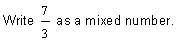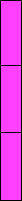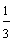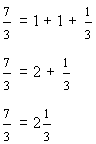In example 5, the improper fraction seven-thirds is written as the mixed number two and one-third, where two is the whole-number part, and one-third is the fractional part.

Example 6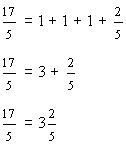In example 6, the improper fraction seventeen-fifths is written as the mixed number three and two-fifths, where three is the whole-number part, and two-fifths is the fractional part.

In example 7, we will write each number using words. We will then classify each number as a proper fraction, an improper fraction, or a mixed number. Place your mouse over the answer text see if you got it right.

 Example 7 Number Words Type of Fraction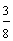three-eighths answer 1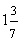one and three-sevenths answer 2five-fifths answer 3eleven-fourths answer 4three and five-sixths answer 5eight-thirds answer 6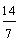fourteen-sevenths answer 7

The last number in example 7 can be written as a whole number: fourteen-sevenths is equal to two wholes (2).

How can you tell if a fraction is less than 1, equal to 1, or greater than 1?

 Compare the numerator and denominator Example Type of Fraction Write As If the numerator <  denominator, then the fraction < 1.proper fraction proper fraction If the numerator = denominator, then the fraction = 1.improper fraction whole number If the numerator > denominator, then the fraction > 1.improper fraction mixed number

Summary: A number can be classified as a proper fraction, an improper fraction, or as a mixed number. Any number divided by itself is equal to one. A mixed number consists of a whole-number part and a fractional part.

Exercises

In Exercises 1 through 5, click once in an ANSWER BOX and type in your answer; then click ENTER. After you click ENTER, a message will appear in the RESULTS BOX to indicate whether your answer is correct or incorrect. To start over, click CLEAR. Note: To write the fraction two-thirds, enter 2/3 into the form.

 1. Write a proper fraction using only the digits 7 and 2. ANSWER BOX:   RESULTS BOX:
 2. Write an improper fraction using only the digits 5 and 8. ANSWER BOX:   RESULTS BOX:
 3. Write twelve-sixths as a whole number. ANSWER BOX:   RESULTS BOX:
 4. Write one and two-thirds as an improper fraction. ANSWER BOX:   RESULTS BOX:
 5. Write one and one-fourth as an improper fraction. ANSWER BOX:   RESULTS BOX:
 Lessons on Fractions 1. Introduction to Fractions 2. Classifying Fractions 3. Equivalent Fractions 4. Simplifying Fractions 5. Comparing Fractions 6. Ordering Fractions 7. Converting Fractions to Mixed Numbers 8. Converting Mixed Numbers to Fractions 9. Practice Exercises 10. Challenge Exercises 11. Solutions